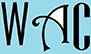## Geometry Question for Title 1 Schools: Third Angle Question?

6) If two angles of a triangle are 48o and 72o, what is the value of the third angle?

The total angle of a triangle   = 180o

Therefore the sum of the other two angles must be subtracted from 180o

= 180o  – (48+ 72o)

180o  – 120o

= 60o